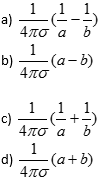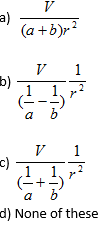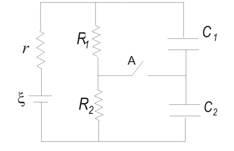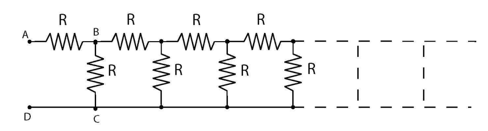Practice Questions on Electric current resistance and resistivity for JEE Main and Advanced

Paragraph Type Questions

Two concentric metal spherical shells of radius a and b respectively are separated by weakly conducting material of conductivity σ
A potential difference V is applied across the two shells
Question 1) Find the resistance between the shellsQuestion 2 Find the electric field at  a distance r from the centerQuestion 3
Consider the figure given belowWhere E=10V is a source of emf with internal resistance r=0.5Ω which is connected to two resistors R1=2Ω and R2=4Ω. Two capacitors C1=0.02µF and C2=0.05µF are connected in parallel to the resistors. Calculate
a)Current in the circuit and charges Q1 and Q­2 on the capacitors when steady state is reached with switch A open.
b)Current in the circuit and charges Q1 and Q­2 on the capacitors when steady state is reached with switch A closed

Question 4
Consider the infinite network of resistors as shown below in the figureEach resistor in the infinite network has a value of 2Ω. Find the current drawn from 12V supply of internal resistance 0.5Ω.

Solutions